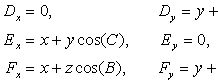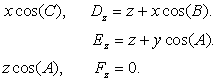##1. Pedal triangle of a point with respect to a triangle

Consider triangle ABC and a point D on its plane. Let {D1,D2,D3} be its projections on the sides of the
triangle. Triangle D1D2D3 is the pedal of D. The signed distances of D from the sides of the triangle are
the trilinear coordinates of the point D. All the elements of the pedal can be expressed in terms of these
trilinears and the sides of the triangle of reference ABC. Below is a compedium of such formulas.
Except the first and the last the other formulas give respective results for the other sides by cyclically
changing the coordinates.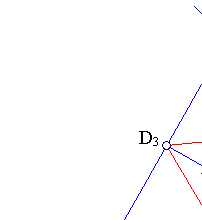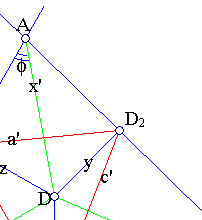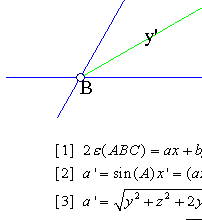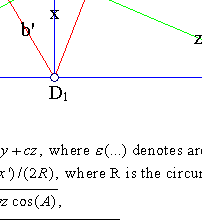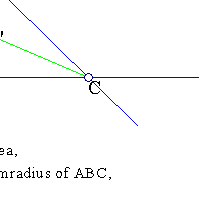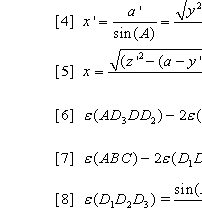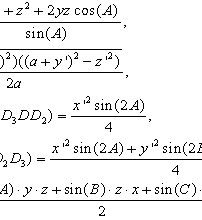results by calculating the area of ABC in terms of (x,y,z).
[2,3] are shown in
CoordinatesBasics.html .
 is a consequence of the previous.
 is a calculation of the altitude of
a triangle.
[6, 7] were shown by Steiner as follows:
Because AD3DD2 is cyclic sin(D2DD3)=sin(A) and the left side of  is:
-DD3*DD2*sin(A)) = (sin(A)*x'2/2)(cos(fi)cos(A-fi)-sin(fi)sin(A-fi)) = (sin(A)x'2/2)*cos(A).
 is a consequence of  and  is proved in AreaOfPedal.html .

##2. Additional properties

The circumcircle (c) of triangle D1D2D3  cuts the sides at three other points correspondingly
{E1,E2,E3}, so that:
 {E1,E2,E3} are the projections of another point E on the sides of ABC.
 Points {D,E} are symmetric with respect to the circumcenter O of D1D2D3 (or/and E1E2E3).
 Points {D,E} are isogonal conjugate with respect to ABC.
 Line AH, H being the intersection of lines {D2D3, E2E3} is orthogonal to DE.
 Line D3E2 passes through the pole F of AH with respect to c.
 The perspectivity axis of point-perspective triangles {DD2D3, EE3E2}, line HIK, passes through the
center O of (c) and is orthogonal to AF.
Analogous statements hold for similar constructs with respect to the other vertices {B, C} of triangle ABC.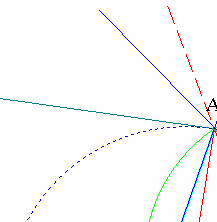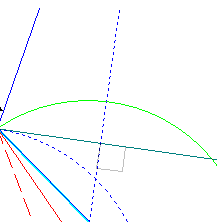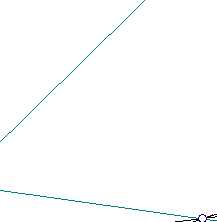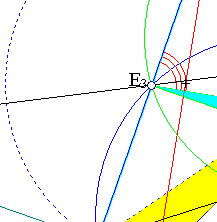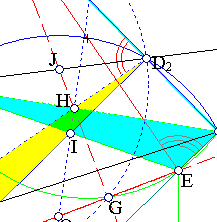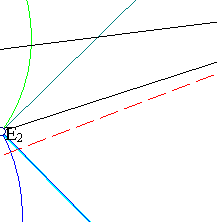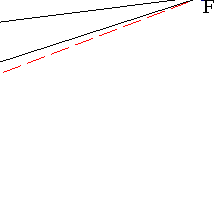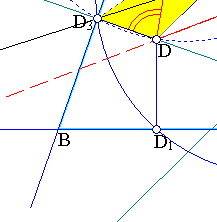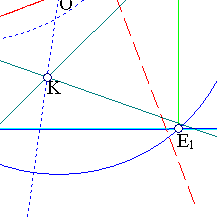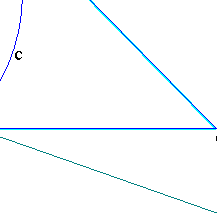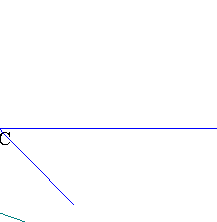,  Consider the projections of O on the sides and identify Ei as the symmetrics of Di.
 Angle chasing as indicated.
 Follows from  by showing the similarity of the corresponding triangles.
 H is on the radical axis of the circumcircles of the similar quadrangles (actually it is the radical
center of the three circles drawn). Since AD, AE are diameters of these circles, their radical axis is
orthogonal to line DE.
 Considering F to be the intersection point of {D2E3, D3E2} we have a complete quadrilateral and F
is the pole of AH with respect to the circle, thus the orthogonal OG to AJ (see ) passes through F.
 Follows from .
 The perspectivity axis is also diagonal of parallelogram DKEI, hence passes through the middle O
of DE. By an argument like that of  we deduce that H is the pole of line AF, hence OH is
orthogonal to AF.

##3. The third pedal

The third pedal t3 = JKL of a triangle t = ABC is similar to ABC.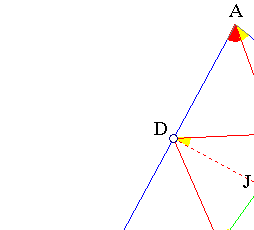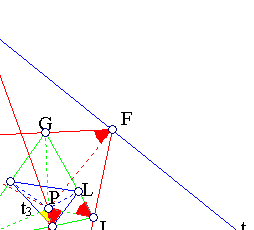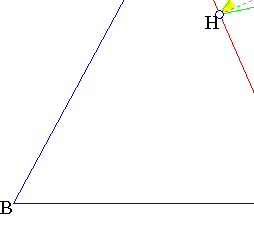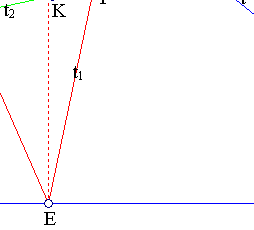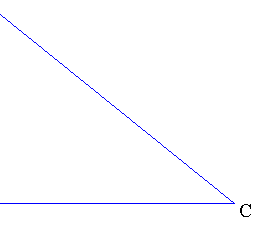The proof is an easy angle chasing as indicated by the picture. Pedal t1=DEF is created by projecting
point P on the sides of t. Pedal t2=GHI (of DEF) is created by projecting P on the sides of t1 etc..

##4. Inscribed triangles

Except trilinears, pedals are connected with other subjects of the euclidean geometry. Among them
the problem of inscribing a triangle into another triangle and the so-called pivots of this
inscription. Projecting namely the point P on the sides of ABC not orthogonally but at an angle (fi)
to the orthogonal we obtain triangles similar to the pedal. We obtain thus a family of similar
triangles A'B'C' of which the pedal one is the smallest in area (or perimeter).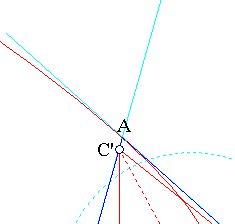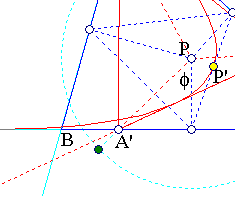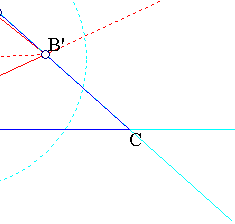This can be easily seen by observing that triangles {PA'B', PB'C', PC'A'} remain similar to
themselves and their sides opposite to P envelope a parabola whose one focus is P and the vertex
is the projection P' of P on a side of the pedal (see ParabolaNewton.html for details on this).

##5. Pedal triangles of particular points

 Pedal of incenter, the triangle formed by the contact points.
 Pedal of circumcenter, formed by the middles of the sides (medial triangle).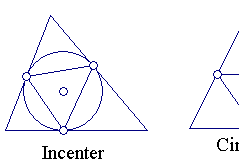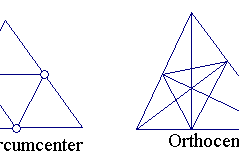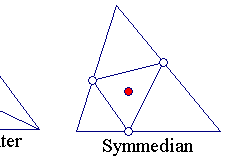Pedal of orthocenter of ABC: orthic triangle of ABC.
 Pedal of symmedian point of ABC: triangle similar to the one formed by the medians of ABC.

##6. Problems

 Show that the orthocenter has the "smallest" pedal (Fagnano's problem).
 Find properties of the pedal of the centroid.
 Characterize points P for which the lines joining their projections to opposite vertices are
concurrent (Rigby pedal cubic).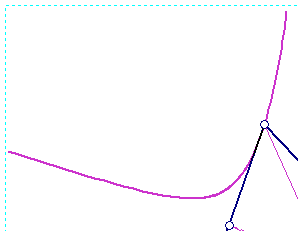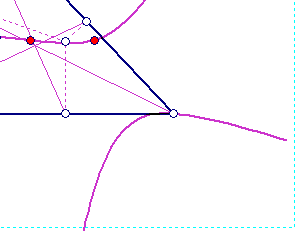The above figure shows the Rigby pedal cubic of the triangle together with some points on it:
the incenter, circumcenter, orthocenter, Gergonne point, and de Longchamps point.

##7. Pedal and Cevian triangles of a point

The pedal and Cevian triangles of a point are two triangles created by simple recipes from a triangle ABC and
a point D. The pedal has for vertices the projections of P on the sides.
The Cevian has for vertices the traces of lines {AP,BP,CP} respectively on the opposite sides.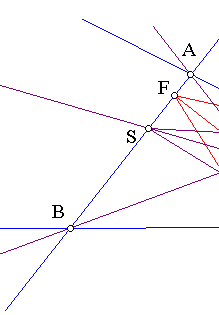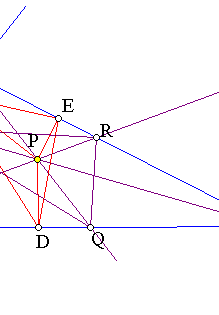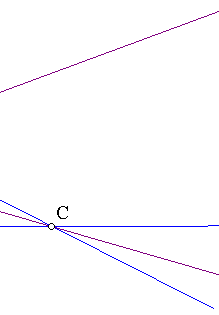The trilinears of the traces {Q,R,S} can be computed easily from the trilinears (x,y,z) of P and the results
obtained in TrilinearsRelatedToOthers.html .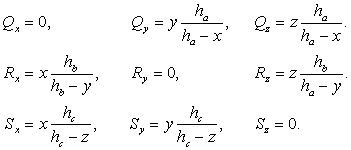Analogously the trilinears of the projections {D,E,F} of P can be easily computed.